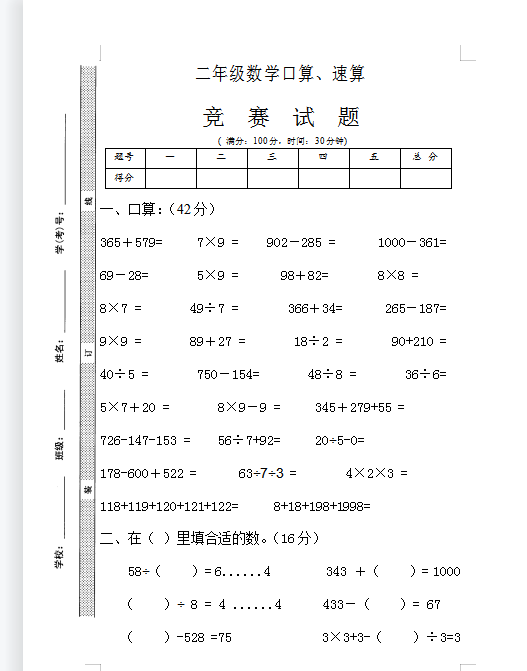( 满分：100分，时间：30分钟)

 题号 一 二 三 四 五 总 分 得分

365＋579=     7×9 =    902－285 =      1000－361=

69－28=       5×9 =      98＋82=       8×8 =

8×7 =       49÷7 =       366＋34=      265－187=

9×9 =       89＋27 =       18÷2 =       90+210 =

40÷5 =       750－154=       48÷8 =       36÷6=